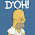## 星期一, 三月 19, 2012

### 如何用21点来击败赌场？

21点也许是世界上最受欢迎的扑克牌游戏之一。玩家要尽量使手中牌的点数和达到21点，或是接近21点，但不能超过，再和庄家比较点数和的大小以定输赢。熟悉概率的诸位都知道，在赌场的各类游戏中，庄家有着绝对的优势。但二十一点却是唯一有机会击败庄家的游戏，也是最需要冷静和计算的游戏。其秘诀就在于记牌和算牌，即玩家要记住所有打出去的牌，然后再决定如何打剩下的牌。

UCLA的数学教授爱德华·索普（Edward Thorp）在六十年代初发明了正式的21点算牌法。那时计算机也发明出来了，他找到IBM公司里的朋友，写了个程序来验证自己的算牌方法。那时的计算机足足运转了七天七夜，终于证明了这个方法是可行的。索普又自己到赌场里亲自实践，果然大赢特赢。1962年他出版了《打败庄家（Beat the Dealer）》一书，向公众介绍了自己的算牌法。《打败庄家》在刚出版时轰动一时，很快成为畅销书，激励了无数赌徒涌向赌场。赌场对此大为恐慌，有些甚至关闭了二十一点赌桌。但是，很快他们发现，只有极少数人真正掌握了算牌法，其他大多数人只不过是一知半解、道听途说。索普本人在60年代后期就淡出了赌博界，带着他在赌场赢来的大笔资金，进入股票市场，运用他的数学知识，现在已成为超级巨富。

R代码如下：
```# 从13张扑克牌中抽一张牌的函数，用n和m来控制大小牌的抽取概率
card.select <- function(n=1,m=6) {
x <- sample(1:13,1,prob=rep(c(n,m),c(9,4))/sum(rep(c(n,m),c(9,4))))
# J，Q，K都被转为10点
x <- ifelse(x>10,10,x)
return(x)
}

# 完成一次二十一点游戏的函数，point为是否继续要牌的判断阀值
game <- function(point) {
# 最开始得到的两张牌
select <- c(card.select(),card.select())
# 将原始序列select中的1点转成11点，求牌的点数和
Ato11 <- select
Ato11[Ato11==1] <- 11
card.sum <- sum(Ato11)
# 进入条件循环，以判断是否应该继续要牌
#若牌点数和大于阀值，则不再要牌
while (card.sum <= point) {
select <- c(select, card.select())
Ato11 <- select
Ato11[Ato11==1] <- 11
card.sum <- sum(Ato11)
}
# 若点数和大于21且其中有A，则A看作1点，用原始的序列求和
if (card.sum > 21 && 1 %in% select) {
card.sum <- sum(select)
}
# A转为1点后，再次条件循环，以判断是否应该继续拿牌
while (card.sum <= point) {
select <- c(select, card.select())
card.sum <- sum(select)
}
# 若点数大于21点，则爆牌为0
y <-ifelse((card.sum<=21),card.sum,0)
return(y)
# cat('select=',select,'\n','return=',y,'\n')
}

# 与庄家博弈，赌场规定庄家以16为阀值，玩家应以12为阀值，等待庄家爆掉
player <- replicate(100000,game(12))
dealer <- replicate(100000,game(16))
#结果有赢输平三种情况
result <-ifelse(player > dealer,1,ifelse(player < dealer,-1,0))
# 若二者均爆牌且平局，设玩家输
result[player==0 & result==0] <- -1
# 将平的情况略去以方便进行二项检验
result.no.tie <- result[result!=0]
# 二项检验
binom.test(length(result.no.tie[result.no.tie==1]),length(result.no.tie))
# 观察玩家连赢或连输的游程
table(rle(result))
# 计算累积利润
profit <- cumsum(result)
# 绘图以观察利润变化
q <- ggplot(data.frame(profit,index=1:length(profit)),aes(index,profit))
q + geom_line(colour='lightskyblue4')

# 考查在不同的大小牌比值的情况下，玩家获胜的概率
odd.10 <-  win <- rep(0,10)
for (i in 1:10) {
card.select <- function(n=1,m=i) {
x <- sample(1:13,1,prob=rep(c(n,m),c(9,4))/sum(rep(c(n,m),c(9,4))))
x <- ifelse(x>10,10,x)
return(x)
}
odd.10[i] <- 4*i/9
player <- replicate(100000,game(12))
dealer <- replicate(100000,game(16))
result <-ifelse(player > dealer,1,ifelse(player < dealer,-1,0))
result[player==0 & result==0] <- -1
result.no.tie <- result[result!=0]
win[i] <- length(result.no.tie[result.no.tie==1])/length(result.no.tie)
}

d <- ggplot(data.frame(odd.10,win),aes(odd.10,win))
d + geom_line(colour='lightskyblue4',size=1) +
geom_point(colour='red4',size=3.5) +
geom_hline(y=0.5,linetype=2)```

#### 9 条评论:

1.非常牛x的文章！现在赌场都会在洗完一副牌之后，先拿出几张（~10）放在一边不用。这样就会大大影响算盘的效率了

1.赌场越来越精罗

2.大哥，最重要还是数学，白人读书都不是为了考试，而为了求真，学概率的求真就赚钱了，比不求真的去赌场输得少。

3.已经有论文把现实中所有条件都考虑到，不过一般人不容易懂。

中文哪有论文研究这个？

4.朋友，厉害！

5.此评论已被作者删除。

6.此评论已被作者删除。

7.现在欧美casino里都用自动洗牌机了，出来的牌直接塞回去，再随机发出来，算牌几乎没用。

8.比特币的投资价值
得到 全球的肯定

股票、外汇、等全球千种金融产品的交易与投资 投资世界最受欢迎的金融市场从未如此简单